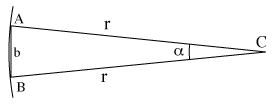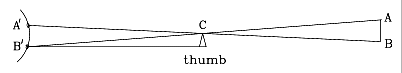# (8b) ParallaxIndex5c. Coordinates6. The Calendar6a. Jewish Calendar7.Precession8. The Round Earth8a. The Horizon8b. Parallax8c. Moon dist. (1)8d. Moon dist. (2)9a. Earth orbits Sun?9c. Copernicus
to Galileo10. Kepler's Laws10a. Scale of Solar Sys.11a. Ellipses
and First Law## "Pre-Trigonometry"

Section M-7 describes the basic problem of trigonometry (drawing on the left): finding the distance to some far-away point C, given the directions at which C appears from the two ends of a measured baseline AB. This problem becomes somewhat simpler if:
1. The baseline is perpendicular to the line from its middle to the object, so that the triangle ABC is symmetric. We will denote its side by r:

AC = BC = r

2. The length c of the baseline AB is much less than r. That means that the angle α between AC and BC is small; that angle is known as the parallax of C, as viewed from AB.

3. We do not ask for great accuracy, but are satisfied with an approximate value of the distance--say, within 1%.
The method presented here was already used by the ancient Greeks more than 2000 years ago. They knew that the length of a circle of radius r was 2πr, where π (a modern notation, not one of the Greeks, even though π is part of their alphabet) stands for a number a little larger than 3, approximately

π = 3.14159...

(The Greek mathematician Archimedes derived π to about 4-figure accuracy, though he expressed it differently, since decimal fractions appeared in Europe only some 1000 years later.)

 Draw a circle around the point C, with radius r, passing through A and B (drawing above). Since the angle α is so small, the length of the straight-line "baseline" b (drawing on the right; distance AB renamed) is not much different from the arc of the circle passing A and B. Let us assume the two are the same (that is the approximation made here). The length of a circular arc is proportional to the angle it covers, and sinceb covers an angle    α
2π r covers an angle 360°

we get

2π r = (360°/ α b)

and dividing by 2π

r = (360°/2 π α) b

Therefore, if we know b, we can deduce r. For instance, if we know that α = 5.73°, 2 π α = 36° and we get

r = 10 b

## Estimating distance outdoors

Here is a method useful to hikers and scouts. Suppose you want to estimate the distance to some distant landmark--e.g. a building, tree or water tower.

The drawing shows a schematic view of the situation from above (not to scale). To estimate the distance to the landmark A, you do the following: